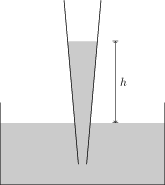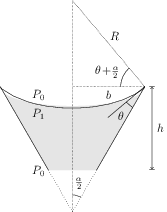# Capillary Rise

## Problems from IIT JEE

Problem (IIT JEE 2014):A glass capillary tube is of the shape of a truncated cone with an apex angle $\alpha$ so that its two ends have cross sections of different radii. When dipped in water vertically, water rises in it to a height $h$, where the radius of its cross-section is $b$. If the surface tension of water is $S$, its density is $\rho$, and its contact angle with glass is $\theta$, the value of $h$ will be (where $g$ is acceleration due to gravity),

1. $\frac{2S}{b\rho g}\cos(\theta-\alpha)$
2. $\frac{2S}{b\rho g}\cos(\theta+\alpha)$
3. $\frac{2S}{b\rho g}\cos(\theta-\alpha/2)$
4. $\frac{2S}{b\rho g}\cos(\theta+\alpha/2)$

Solution:Let $R$ be the radius of the meniscus formed with a contact angle $\theta$ (see figure). By geometry, this radius makes an angle $\theta+\frac{\alpha}{2}$ with the horizontal and, \begin{align} \label{oxb:eqn:1} \cos\left(\theta+\tfrac{\alpha}{2}\right)={b}/{R}. \end{align} Let $P_0$ be the atmospheric pressure and $P_1$ be the pressure just below the meniscus. Excess pressure on the concave side of meniscus of radius $R$ is, \begin{align} \label{oxb:eqn:2} P_0-P_1={2S}/{R}. \end{align} The hydrostatic pressure gives, \begin{align} \label{oxb:eqn:3} P_0-P_1=h\rho g. \end{align} Eliminate $(P_0-P)$ from second and third equations and substitute $R$ from first equation to get, \begin{align} h=\frac{2S}{\rho g R}=\frac{2S}{b\rho g}\cos\left(\theta+\tfrac{\alpha}{2}\right).\nonumber \end{align}